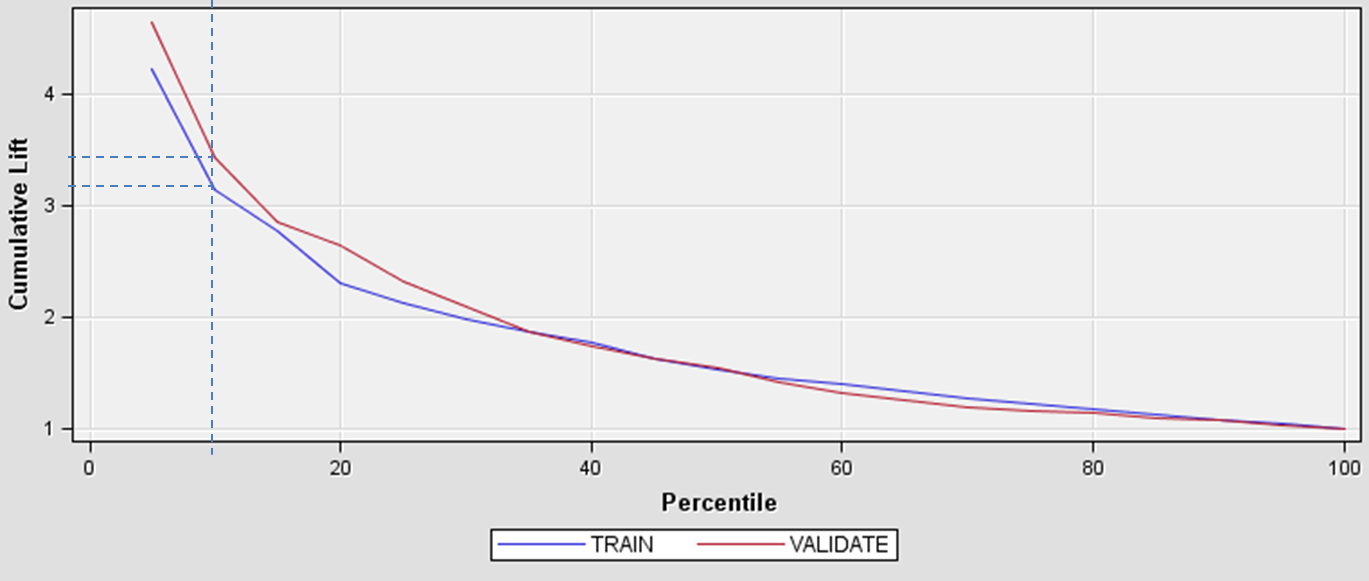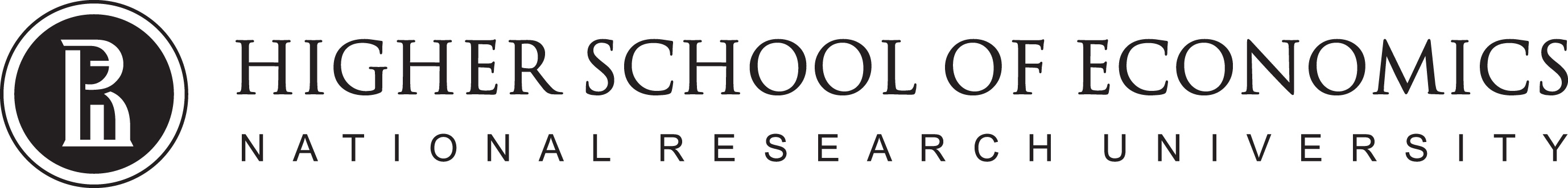# Data Analysis

## Supervised learning quality measures1

1. Some materials are taken from machine learning course of Victor Kitov

# Let's recall previous lecture¶

• Linear Classification
• Binary linear classifier: $\widehat{y}(x)=sign(w^{T}x+w_{0})$.
• Various multiclassification approaches: 1-vs-all, 1-vs-1, etc..
• Perceptron, logistic, SVM - linear classifiers estimated with different loss functions.
• Optimized with gradient descent

# Model Evaluation¶

• If we have several models we need to understand which one is better then the other
• If performance of complex model is similar to performance of more simplistic model use the latter!
• Understand the profit of our model in business domain (see this)
• Check if ML model is performind better then simple heuristics or (almost) zero-information prediction, or simple baseline models
• Always predict the majority class (classification)
• Always predict the mean value (regression)
• Build a simple model and compare it's performance with your sophisticated model

## Before we go¶

### Recall the difference between loss function and quality measures¶

• Loss functions are directly optimized by the algorithms
• Loss functions allways can be computed for a single object
• Quality measures are usually computed for a set

# Quality measures: Regression¶

1. (R)MSE ((Root) Mean Squared Error)

$$L(\hat{y}, y) = \frac{1}{N}\sum\limits_{n=1}^N (y_n - \hat{y}_n)^2$$

2. MAE (Mean Absolute Error)

$$L(\hat{y}, y) = \frac{1}{N}\sum\limits_{n=1}^N |y_n - \hat{y}_n|$$
• What are key differences?
• What are key issues?

## MAE and MSE¶

• Different scales
• MSE penalize greater error more
• MAE is robust to outliers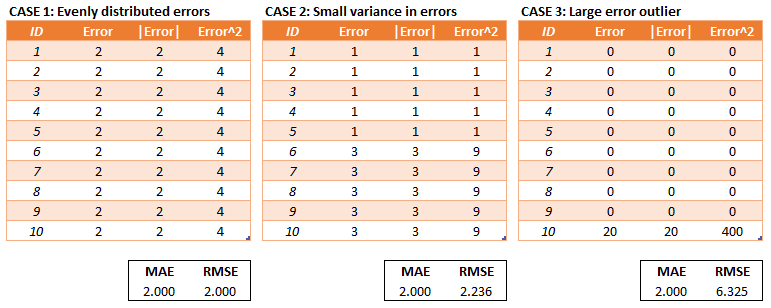• We can compare models with MAE and MSE but it is hard to tell if a model is good overall...

3. RSE (Relative Squared Error)

$$L(\hat{y}, y) = \sqrt\frac{\sum\limits_{n=1}^N (y_n - \hat{y}_n)^2}{\sum\limits_{n=1}^N (y_n - \bar{y})^2}$$

4. RAE (Relative Absolute Error)

$$L(\hat{y}, y) = \frac{\sum\limits_{n=1}^N |y_n - \hat{y}_n|}{\sum\limits_{n=1}^N |y_n - \bar{y}|}$$

5. MAPE (Mean Absolute Persentage Error)

$$L(\hat{y}, y) = \frac{100}{N} \sum\limits_{n=1}^N\left|\frac{ y_n - \hat{y}_n}{y_n}\right|$$

6. RMSLE (Root Mean Squared Logarithmic Error)

$$L(\hat{y}, y) = \sqrt{\frac{1}{N}\sum\limits_{n=1}^N(\log(y_n + 1) - \log(\hat{y}_n + 1))^2}$$
• what is so special about it?
In :
y = 10000
y_hat = np.linspace(0, 30000, 151)
# log error
error1 = np.sqrt((np.log(y+1) - np.log(y_hat + 1))**2)

# squared error
error2 = (y - y_hat)**2 /1000.

plt.plot(y_hat, error1, label='RMSLE')
plt.plot(y_hat, error2, label='MSE')
plt.xlabel('$\hat{y}$')
plt.ylabel('Error')
plt.title('true value y = %.1f' % y)
plt.legend()
plt.ylim(0, 10)

Out:
(0, 10)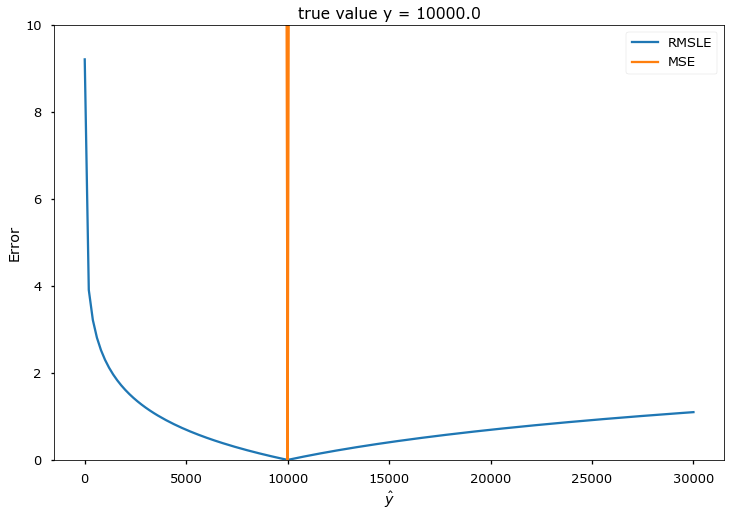# Quality measures: Classification¶

## Confusion matrix¶

Confusion matrix $M=\{m_{ij}\}_{i,j=1}^{C}$ shows the number of $\omega_{i}$ class objects predicted as belonging to class $\omega_{j}$.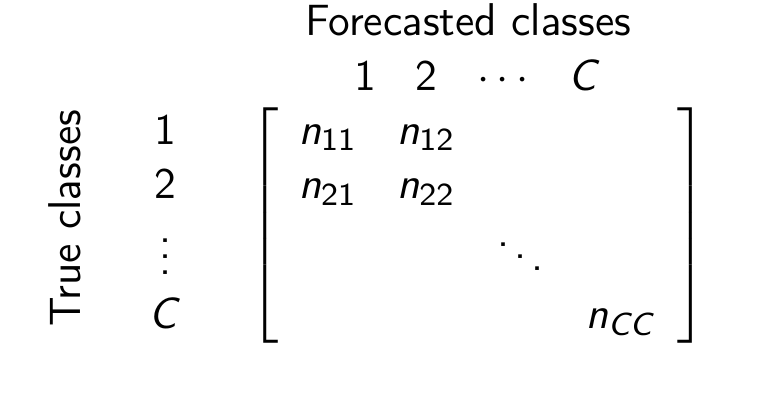Diagonal elements correspond to correct classifications and off-diagonal elements - to incorrect classifications.

## Confusion matrix¶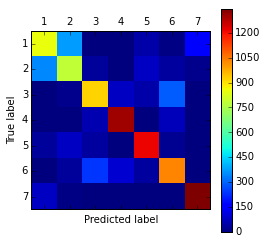• We see here that errors are conсentrated between classes 1 and 2
• We can
• unite classes 1 and 2 into class "1+2"
• solve 6-class classification problem (instead of 7)
• try to separate classes 1 and 2 afterwards

## 2 classes case¶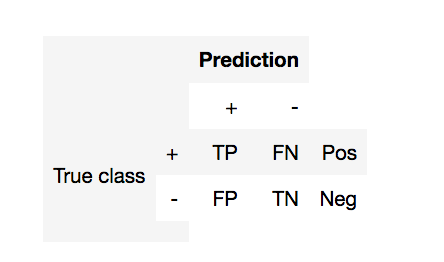• TP (true positive) - currectly predicted positives
• FP (false positive) - incorrectly predicted negatives (1st order error)
• FN (false negative) - incorrectly predicted positives (2nd order error)
• TN (true negative) - currectly predicted negatives
• Pos (Neg) - total number of positives (negatives)
• Provide examples of task with positive classes and negative classes
• Why do you define them in that way and not another?

## 2 classes case¶• $\text{accuracy} = \frac{TP + TN}{Pos+Neg}$
• $\text{error rate} = 1 -\text{accuracy}$
• $\text{precision} =\frac{TP}{TP + FP}$ - (точность)
• Ratio of objects marked positive are actually positive
• $\text{recall} =\frac{TP}{TP + FN} = \frac{TP}{Pos}$ - (полнота)
• Ratio of positive objects currectly classified
• $\text{F}_\beta \text{-score} = (1 + \beta^2) \cdot \frac{\mathrm{precision} \cdot \mathrm{recall}}{(\beta^2 \cdot \mathrm{precision}) + \mathrm{recall}}$
• why harmonic mean?
• What about multiclassification case?
In :
fig = interact(demo_fscore, beta=FloatSlider(min=0.1, max=5, step=0.3, value=1))


## Discriminant decision rules¶

• Decision rule based on discriminant functions:

• predict $\omega_{1}$ $\Longleftrightarrow$ $g_{1}(x)-g_{2}(x)>\mu$
• predict $\omega_{1}$ $\Longleftrightarrow$ $g_{1}(x)/g_{2}(x)>\mu$ (for $g_{1}(x)>0,\,g_{2}(x)>0$)
• Decision rule based on probabilities:

• predict $\omega_{1}$ $\Longleftrightarrow$$P(\omega_{1}|x)>\mu ## Class label versus class probability evaluation¶ • Discriminability quality measures evaluate class label prediction. • examples: error rate, precision, recall, etc.. • Reliability quality measures evaluate class probability prediction. • Example: probability likelihood:$$ \prod_{n=1}^{N}\widehat{p}(y_{n}|x_{n}) $$• Brier score:$$ \frac{1}{N}\sum_{n=1}^{N}\sum_{c=1}^{C}\left(\mathbb{I}[y_{n}=c]-\widehat{p}(y=c|x_{n})\right)^{2} $$• Logloss (cross entropy):$$ \frac{1}{N}\sum_{n=1}^{N}\sum_{c=1}^{C}\mathbb{I}[y_{n}=c]\ln(\widehat{p}(y=c|x_{n})) $$## ROC curve¶ • ROC curve - is a function TPR(FPR). • It shows how the probability of correct classification on positive classes ("recognition rate") changes with probability of incorrect classification on negative classes ("false alarm"). • It is build as a set of points TPR(\mu), FPR(\mu). • If \mu \downarrow , the algorithm predicts \omega_{1} more often and • TPR=1-\varepsilon_{1} \uparrow • FPR=\varepsilon_{2} \uparrow • Characterizes classification accuracy for different \mu. • more concave ROC curves are better • TPR = \frac{TP}{TP + FN}=\frac{TP}{Pos} • FPR = \frac{FP}{FP + TN} = \frac{FP}{Neg}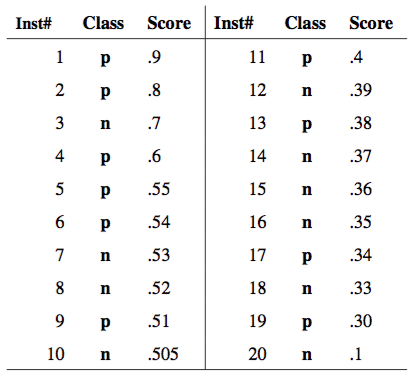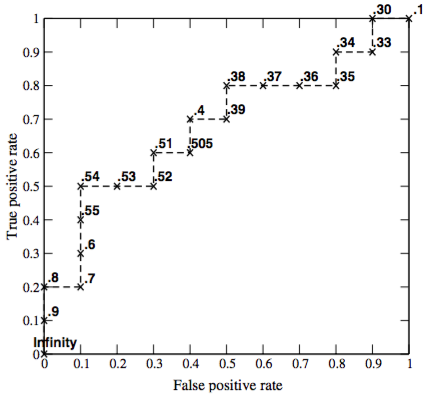## How to compare ROCs?¶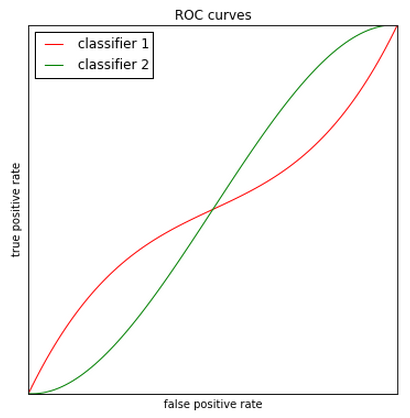## ROC-AUC¶ • Area under the ROC curve • Global quality characteristic for different \mu • AUC\in[0,1] • AUC = 0.5 - equivalent to random guessing • AUC = 1 - no errors classification. • AUC property: it is equal to probability that for 2 random objects x_{1}\in + and x_{2}\in - it will hold that: \widehat{p}(+|x_{1})>\widehat{p}(+|x_2) • What about unbalanced case? ## Precision Recall Curve¶ • Build in the same manner as ROC curve • Consider computing PR-AUC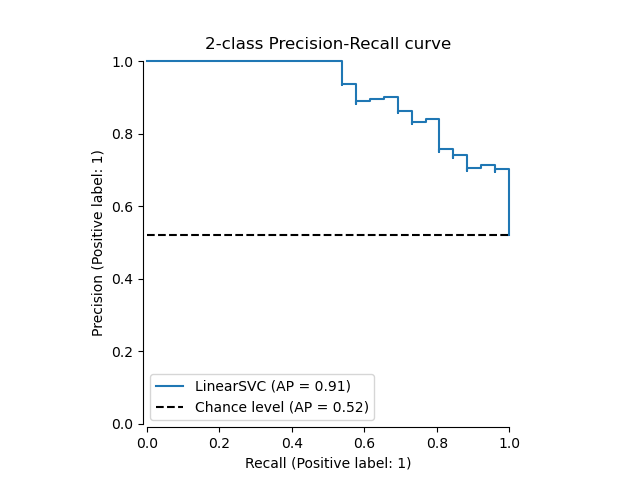## Model Lift¶ • Let r_{POS} - positive class rate in the whole dataset • Let TPR @ K\% be positive class rate in top K \% segment of the dateset, sorted by score$$ Model Lift @ K\% = \frac{TPR @ K\%}{r_{POS}}$\$